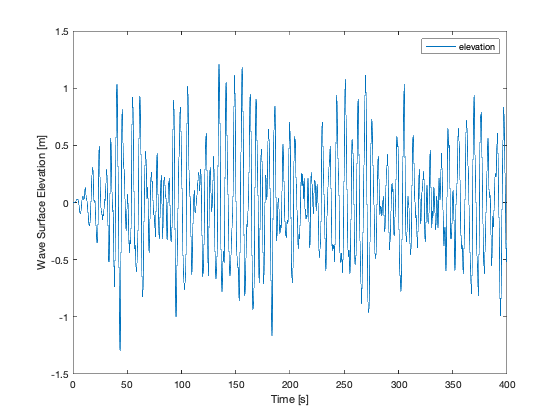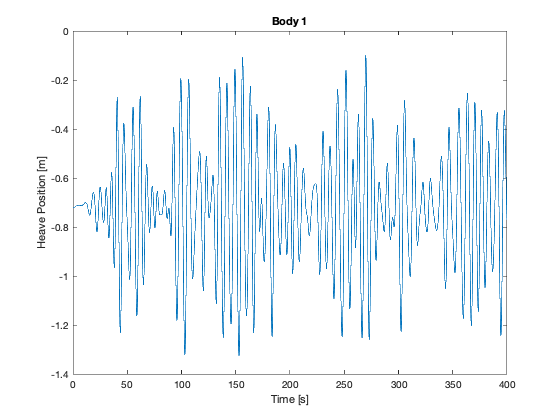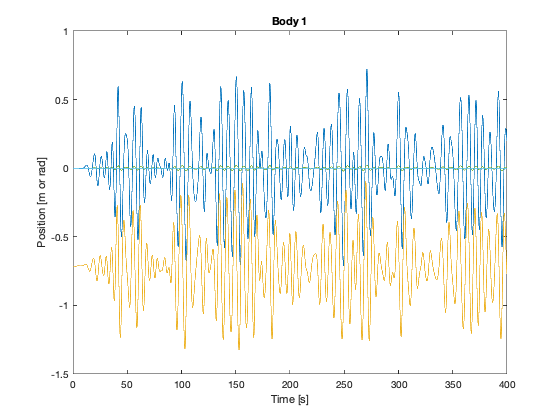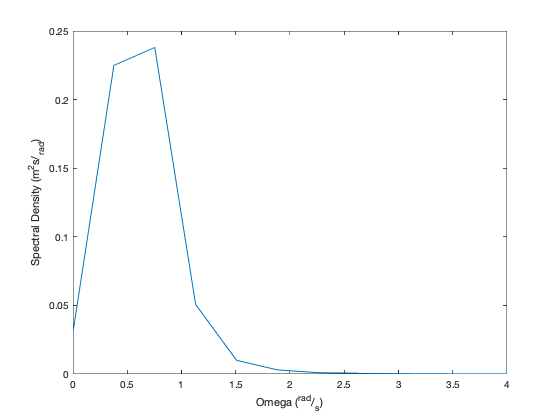# Example: MHKiT-MATLAB WEC-Sim

This example loads simulated data from a WEC-Sim run for a two-body point absorber (Reference Model 3) and demonstrates the application of the MHKiT Wave Module to interact with the simulated data. The analysis is broken down into three parts: load WEC-Sim data, interact with the WEC-Sim data, and apply the MHKiT wave module.
2. Analyze WEC-Sim Simulated Data (e.g. Wave Class, Body Class, PTO Class, Constraint Class, and Mooring Class Data)
3. Apply MHKiT Wave Module

## 1. Load WEC-Sim Simulated Data

WEC-Sim saves output data as a MATLAB structure, generated by WEC-Sim’s Response Class. The WEC-Sim structure contains data for: wave, bodies, ptos, constraints, mooring, moordyn, and ptosim
Here we will load the WEC-Sim RM3 data run with a mooring matrix.
filename = './data/RM3MooringMatrix_matlabWorkspace.mat'
filename = './data/RM3MooringMatrix_matlabWorkspace.mat'
output
output = struct with fields:
wave: [1×1 struct] bodies: [1×2 struct] ptos: [1×1 struct] constraints: [1×1 struct] mooring: [1×1 struct] moorDyn: [1×1 struct] ptosim: [1×1 struct]

## 2. Analyze WEC-Sim Simulated Data

This section will analyze the WEC-Sim RM3 data using the WEC-Sim structure.

### Wave Class Data

Data from WEC-Sim’s Wave Class includes information about the wave input, including the the wave type, and wave elevation as a function of time.
% Store WEC-Sim output from the Wave Class to a new variable, called `wave_data`
wave_data = output.wave
wave_data = struct with fields:
type: 'etaImport' time: [40001×1 double] elevation: [40001×1 double]
figure;plot(wave_data.time,wave_data.elevation)
xlabel("Time [s]")
ylabel("Wave Surface Elevation [m]")
legend('elevation')### Body Class Data

Data from WEC-Sim’s Body Class includes information about each body, including the body’s position, velocity, acceleration, forces acting on the body, and the body’s name. For the RM3 example there will be 2 boides a float and a spar.
% Store WEC-Sim output from the Body Class as 'bodies'
bodies = output.bodies
bodies = 1×2 struct
1'float'40001×1 double40001×6 double40001×6 double40001×6 double40001×6 double40001×6 double40001×6 double40001×6 double40001×6 double40001×6 double40001×6 double
2'spar'40001×1 double40001×6 double40001×6 double40001×6 double40001×6 double40001×6 double40001×6 double40001×6 double40001×6 double40001×6 double40001×6 double
% Store Body Class data for Body 1 as `body1`.
body1 = output.bodies(1)
body1 = struct with fields:
name: 'float' time: [40001×1 double] position: [40001×6 double] velocity: [40001×6 double] acceleration: [40001×6 double] forceTotal: [40001×6 double] forceExcitation: [40001×6 double] forceRadiationDamping: [40001×6 double] forceAddedMass: [40001×6 double] forceRestoring: [40001×6 double] forceMorrisonAndViscous: [40001×6 double] forceLinearDamping: [40001×6 double]

### Plot heave position data for Body 1

The RM3 device creates power in the heave direction (DOF 3). Let us consider the float's (body 1) position as a function of time by plotting body1.position(:,3).
figure;plot(body1.time, body1.position(:,3))
xlabel("Time [s]")
ylabel("Heave Position [m]")
title('Body 1')% Calculate the maximum and minimum heave position of Body 1
fprintf("Body 1 max heave position = %4.5f [m]", max(body1.position(:,3)))
Body 1 max heave position = -0.09745 [m]
fprintf("Body 1 max heave position = %4.5f [m]", min(body1.position(:,3)))
Body 1 max heave position = -1.32645 [m]
Plot Body 1 position data for all DOFs
As an example we could plot multiple postion DOFs by calling a plot on only the position data.
figure;plot(body1.time, body1.position(:,:))
xlabel("Time [s]")
title('Body 1')## 3. Apply MHKiT Wave Module

In the example below we will calculate a spectrum from the wecsim wave elevation timeseries data using the MHKiT elevation_spectrum function.
% Use the MHKiT Wave Module to calculate the wave spectrum from the WEC-Sim Wave Class Data
sample_rate=60
sample_rate =
60
nnft=1000 % Number of bins in the Fast Fourier Transform
nnft =
1000
ws_spectrum = elevation_spectrum(wave_data.elevation, sample_rate, nnft,wave_data.time)
ws_spectrum = struct with fields:
spectrum: [501×1 double] type: 'Spectra from Timeseries' frequency: [501×1 double] sample_rate: 60 nnft: 1000
% Plot calculated wave spectrum
spect_plot = plot_spectrum(ws_spectrum);
xlim([0, 4]);% Calculate Peak Wave Period (Tp) and Significant Wave Height (Hm0)
Tp = peak_period(ws_spectrum)
8.333333333333334
Tp =
8.333333333333334
Hm0 = significant_wave_height(ws_spectrum)
Hm0 =
1.785739037962701